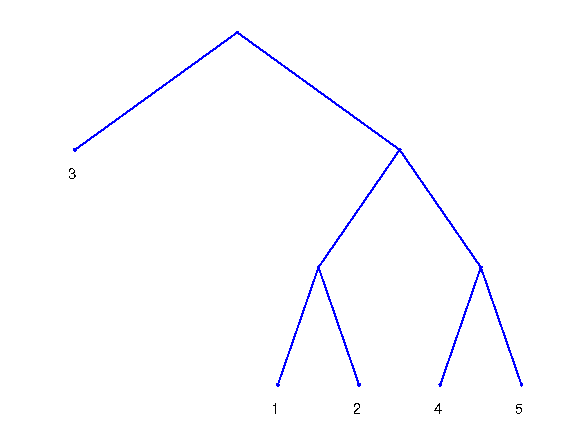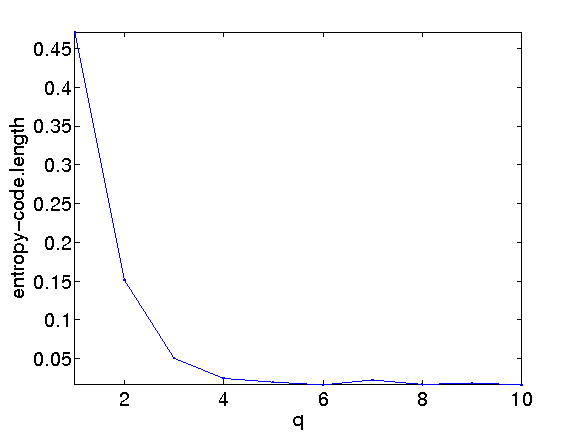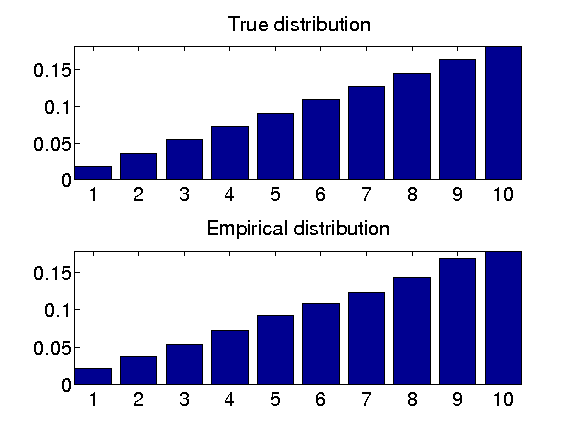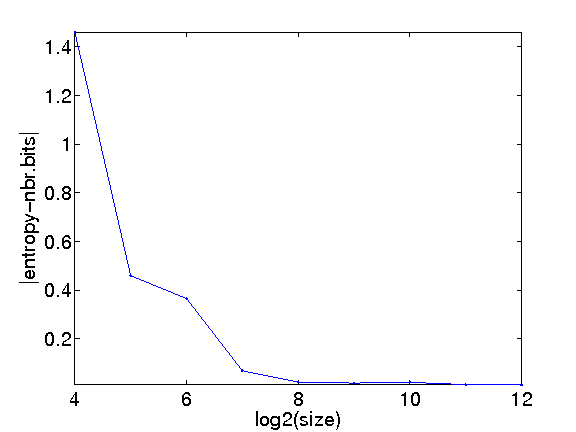# Entropic Coding and Compression¶

Important: Please read the installation page for details about how to install the toolboxes. $\newcommand{\dotp}{\langle #1, #2 \rangle}$ $\newcommand{\enscond}{\lbrace #1, #2 \rbrace}$ $\newcommand{\pd}{ \frac{ \partial #1}{\partial #2} }$ $\newcommand{\umin}{\underset{#1}{\min}\;}$ $\newcommand{\umax}{\underset{#1}{\max}\;}$ $\newcommand{\umin}{\underset{#1}{\min}\;}$ $\newcommand{\uargmin}{\underset{#1}{argmin}\;}$ $\newcommand{\norm}{\|#1\|}$ $\newcommand{\abs}{\left|#1\right|}$ $\newcommand{\choice}{ \left\{ \begin{array}{l} #1 \end{array} \right. }$ $\newcommand{\pa}{\left(#1\right)}$ $\newcommand{\diag}{{diag}\left( #1 \right)}$ $\newcommand{\qandq}{\quad\text{and}\quad}$ $\newcommand{\qwhereq}{\quad\text{where}\quad}$ $\newcommand{\qifq}{ \quad \text{if} \quad }$ $\newcommand{\qarrq}{ \quad \Longrightarrow \quad }$ $\newcommand{\ZZ}{\mathbb{Z}}$ $\newcommand{\CC}{\mathbb{C}}$ $\newcommand{\RR}{\mathbb{R}}$ $\newcommand{\EE}{\mathbb{E}}$ $\newcommand{\Zz}{\mathcal{Z}}$ $\newcommand{\Ww}{\mathcal{W}}$ $\newcommand{\Vv}{\mathcal{V}}$ $\newcommand{\Nn}{\mathcal{N}}$ $\newcommand{\NN}{\mathcal{N}}$ $\newcommand{\Hh}{\mathcal{H}}$ $\newcommand{\Bb}{\mathcal{B}}$ $\newcommand{\Ee}{\mathcal{E}}$ $\newcommand{\Cc}{\mathcal{C}}$ $\newcommand{\Gg}{\mathcal{G}}$ $\newcommand{\Ss}{\mathcal{S}}$ $\newcommand{\Pp}{\mathcal{P}}$ $\newcommand{\Ff}{\mathcal{F}}$ $\newcommand{\Xx}{\mathcal{X}}$ $\newcommand{\Mm}{\mathcal{M}}$ $\newcommand{\Ii}{\mathcal{I}}$ $\newcommand{\Dd}{\mathcal{D}}$ $\newcommand{\Ll}{\mathcal{L}}$ $\newcommand{\Tt}{\mathcal{T}}$ $\newcommand{\si}{\sigma}$ $\newcommand{\al}{\alpha}$ $\newcommand{\la}{\lambda}$ $\newcommand{\ga}{\gamma}$ $\newcommand{\Ga}{\Gamma}$ $\newcommand{\La}{\Lambda}$ $\newcommand{\si}{\sigma}$ $\newcommand{\Si}{\Sigma}$ $\newcommand{\be}{\beta}$ $\newcommand{\de}{\delta}$ $\newcommand{\De}{\Delta}$ $\newcommand{\phi}{\varphi}$ $\newcommand{\th}{\theta}$ $\newcommand{\om}{\omega}$ $\newcommand{\Om}{\Omega}$

This numerical tour studies source coding using entropic coders (Huffman and arithmetic).

In :
warning off
warning on


## Source Coding and Entropy¶

Entropic coding converts a vector |x| of integers into a binary stream |y|. Entropic coding exploits the redundancies in the statistical distribution of the entries of |x| to reduce as much as possible the size of |y|. The lower bound for the number of bits |p| of |y| is the Shannon bound |p=-sum_i h(i)*log2(h(i))|, where |h(i)| is the probability of apparition of symbol |i| in |x|.

Fist we generate a simple binary signal |x| so that 0 has a probability of appearance of |p|.

probability of 0

In :
p = 0.1;


size

In :
n = 512;


signal, should be with token 1,2

In :
x = (rand(n,1)>p)+1;


One can check the probabilities by computing the empirical histogram.

In :
h = hist(x, [1 2]);
h = h/sum(h);
disp(strcat(['Empirical p=' num2str(h(1)) '.']));

Empirical p=0.085938.


We can compute the entropy of the distribution represented as a vector |h| of proability that should sum to 1. We take a |max| to avoid problem with 0 probabilties.

In :
e = - sum( h .* log2( max(h,1e-20) ) );
disp( strcat(['Entropy=' num2str(e)]) );

Entropy=0.42276


## Huffman Coding¶

A Hufman code |C| associate with each symbol |i| in |{1,...,m}| a binary code |C{i}| whose length |length(C{i})| is as close as possible to the optimal bound |-log2(h(i))|, where |h(i)| is the probability of apparition of the symbol |i|.

We select a set of proabilities.

In :
h = [.1 .15 .4 .15 .2];


The tree |T| cotainins the codes is generated by an iterative algorithm. The initial "tree" is a collection of empty trees, pointing to the symbols numbers.

In :
m = length(h);
T = cell(0); % create an empty cell
for i=1:m
T = cell_set(T,i,i);
end


We build iteratively the Huffman tree by grouping together the two Trees that have the smallest probabilities. The merged tree has a probability which is the sums of the two selected probabilities.

initial probability.

In :
p = h;


iterative merging of the leading probabilities

In :
while length(p)>1
% sort in decaying order the probabilities
[v,I] = sort(p);
if v(1)>v(length(v))
v = reverse(v); I = reverse(I);
end
q = sum(v(1:2));
t = cell_sub(T, I(1:2));
% trimed tree
T = cell_sub(T, I(3:length(I)) );
p = v(3:length(v));
% add a new node with the corresponding probability
p(length(p)+1) = q;
T = cell_set(T, length(p), t);
end


We display the computed tree.

In :
clf;
plot_hufftree(T);Once the tree |T| is computed, one can compute the code |C{i}| associated to each symbol |i|. This requires to perform a deep first search in the tree and stop at each node. This is a little tricky to implement in Matlab, so you can use the function |huffman_gencode|.

In :
C = huffman_gencode(T);


display the code

In :
for i=1:size(C,1)
disp(strcat(['Code of token ' num2str(i) ' = ' num2str( cell_get(C,i) )]));
end

Code of token 1 = 1  0  0


We draw a vector |x| according to the distribution h

size of the signal

In :
n = 1024;


randomization

In :
x = rand_discr(h, n);
x = x(:);


Exercise 1

Implement the coding of the vector |x| to obtain a binary vector |y|, which corresponds to replacing each sybmol |x(i)| by the code |C{x(i)}|.

In :
exo1()

In :
%% Insert your code here.


Compare the length of the code with the entropy bound.

In :
e = - sum( h .* log2( max(h,1e-20) ) );
disp( strcat(['Entropy bound = ' num2str(n*e) '.']) );
disp( strcat(['Huffman code  = ' num2str(length(y)) '.']) );

Entropy bound = 2197.9539.
Huffman code  = 2220.


Decoding is more complicated, since it requires parsing iteratively the tree |T|.

initial pointer on the tree: on the root

In :
t = cell_get(T,1);


initial empty decoded stream

In :
x1 = [];


initial stream buffer

In :
y1 = y;
while not(isempty(y1))
% go down in the tree
if y1(1)==0
t = cell_get(t,1);
else
t = cell_get(t,2);
end
% remove the symbol from the stream buffer
y1(1) = [];
if not(iscell(t))
% we are on a leaf of the tree: output symbol
x1 = [x1 t];
t = cell_get(T,1);
end
end
x1 = x1(:);


We test if the decoding is correct.

In :
err = norm(x-x1);
disp( strcat(['Error (should be 0)=' num2str(err) '.']) );

Error (should be 0)=0.


## Huffman Block Coding¶

A Huffman coder is inefficient because it can distribute only an integer number of bit per symbol. In particular, distribution where one of the symbol has a large probability are not well coded using a Huffman code. This can be aleviated by replacing the set of |m| symbols by |m^q| symbols obtained by packing the symbols by blocks of |q| (here we use |m=2| for a binary alphabet). This breaks symbols with large probability into many symbols with smaller proablity, thus approaching the Shannon entropy bound.

Generate a binary vector with a high probability of having 1, so that the Huffman code is not very efficient (far from Shanon bound).

proability of having 1

In :
t = .12;


probability distriution

In :
h = [t; 1-t];


generate signal

In :
n = 4096*2;
x = (rand(n,1)>t)+1;


For block of length |q=3|, create a new vector by coding each block with an integer in |1,...,m^q=2^3|. The new length of the vector is |n1/q| where |n1=ceil(n/q)*q|.

block size

In :
q = 3;


maximum token value

In :
m = 2;


new size

In :
n1 = ceil(n/q)*q;


new vector

In :
x1 = x;
x1(length(x1)+1:n1) = 1;
x1 = reshape(x1,[q n1/q]);
[Y,X] = meshgrid(1:n1/q,0:q-1);
x1 = sum( (x1-1) .* (m.^X), 1 )' + 1;


We generate the probability table |H| of |x1| that represents the probability of each new block symbols in |1,...,m^q|.

In :
H = h;
for i=1:q-1
Hold = H;
H = [];
for i=1:length(h)
H = [H; Hold*h(i)];
end
end


A simpler way to compute this block-histogram is to use the Kronecker product.

In :
H = h;
for i=1:q-1
H = kron(H,h);
end


Exercise 2

For various values of block size |k|, Perform the hufman coding and compute the length of the code. Compare with the entropy lower bound. ntropy bound

In :
exo2()

Entropy=0.52936.
Huffman(block size 1)=1
Huffman(block size 2)=0.68066
Huffman(block size 3)=0.57996
Huffman(block size 4)=0.55396
Huffman(block size 5)=0.54919
Huffman(block size 6)=0.54578
Huffman(block size 7)=0.552
Huffman(block size 8)=0.54626
Huffman(block size 9)=0.54785
Huffman(block size 10)=0.5459In :
%% Insert your code here.


## Arithmetic Coding¶

A block coder is able to reach the Shannon bound, but requires the use of many symbols, thus making the coding process slow and memory intensive. A better alternative is the use of an arithmetic coder, that encode a stream using an interval.

Note : for this particular implementation of an arithmetic coder, the entries of this binary stream are packed by group of 8 bits so that each |y(i)| is in [0,255].

Generate a random binary signal.

probability of 0

In :
p = 0.1;


size

In :
n = 512;


signal, should be with token 1,2

In :
x = (rand(n,1)>p)+1;


The coding is performed using the function |perform_arith_fixed|.

probability distribution

In :
h = [p 1-p];


coding

In :
y = perform_arith_fixed(x,h);

[Warning: BITCMP(A,N) will not accept integer valued input N in a future
[> In perform_arith_fixed>arithenco at 117
In perform_arith_fixed at 23
In pymat_eval at 38
In matlabserver at 27]
[Warning: BITCMP(A,N) will not accept integer valued input N in a future
[> In perform_arith_fixed>arithenco at 117
In perform_arith_fixed at 23
In pymat_eval at 38
In matlabserver at 27]
[Warning: BITCMP(A,N) will not accept integer valued input N in a future
[> In perform_arith_fixed>arithenco at 117
In perform_arith_fixed at 23
In pymat_eval at 38
In matlabserver at 27]
[Warning: BITCMP(A,N) will not accept integer valued input N in a future
[> In perform_arith_fixed>arithenco at 117
In perform_arith_fixed at 23
In pymat_eval at 38
In matlabserver at 27]
[Warning: BITCMP(A,N) will not accept integer valued input N in a future
[> In perform_arith_fixed>arithenco at 117
In perform_arith_fixed at 23
In pymat_eval at 38
In matlabserver at 27]
[Warning: BITCMP(A,N) will not accept integer valued input N in a future
[> In perform_arith_fixed>arithenco at 117
In perform_arith_fixed at 23
In pymat_eval at 38
In matlabserver at 27]
[Warning: BITCMP(A,N) will not accept integer valued input N in a future
[> In perform_arith_fixed>arithenco at 117
In perform_arith_fixed at 23
In pymat_eval at 38
In matlabserver at 27]
[Warning: BITCMP(A,N) will not accept integer valued input N in a future
[> In perform_arith_fixed>arithenco at 117
In perform_arith_fixed at 23
In pymat_eval at 38
In matlabserver at 27]
[Warning: BITCMP(A,N) will not accept integer valued input N in a future
[> In perform_arith_fixed>arithenco at 117
In perform_arith_fixed at 23
In pymat_eval at 38
In matlabserver at 27]
[Warning: BITCMP(A,N) will not accept integer valued input N in a future
[> In perform_arith_fixed>arithenco at 117
In perform_arith_fixed at 23
In pymat_eval at 38
In matlabserver at 27]
[Warning: BITCMP(A,N) will not accept integer valued input N in a future
[> In perform_arith_fixed>arithenco at 117
In perform_arith_fixed at 23
In pymat_eval at 38
In matlabserver at 27]
[Warning: BITCMP(A,N) will not accept integer valued input N in a future
[> In perform_arith_fixed>arithenco at 117
In perform_arith_fixed at 23
In pymat_eval at 38
In matlabserver at 27]
[Warning: BITCMP(A,N) will not accept integer valued input N in a future
[> In perform_arith_fixed>arithenco at 117
In perform_arith_fixed at 23
In pymat_eval at 38
In matlabserver at 27]
[Warning: BITCMP(A,N) will not accept integer valued input N in a future
[> In perform_arith_fixed>arithenco at 117
In perform_arith_fixed at 23
In pymat_eval at 38
In matlabserver at 27]
[Warning: BITCMP(A,N) will not accept integer valued input N in a future
[> In perform_arith_fixed>arithenco at 117
In perform_arith_fixed at 23
In pymat_eval at 38
In matlabserver at 27]
[Warning: BITCMP(A,N) will not accept integer valued input N in a future
[> In perform_arith_fixed>arithenco at 117
In perform_arith_fixed at 23
In pymat_eval at 38
In matlabserver at 27]
[Warning: BITCMP(A,N) will not accept integer valued input N in a future
[> In perform_arith_fixed>arithenco at 117
In perform_arith_fixed at 23
In pymat_eval at 38
In matlabserver at 27]
[Warning: BITCMP(A,N) will not accept integer valued input N in a future
[> In perform_arith_fixed>arithenco at 117
In perform_arith_fixed at 23
In pymat_eval at 38
In matlabserver at 27]
[Warning: BITCMP(A,N) will not accept integer valued input N in a future
[> In perform_arith_fixed>arithenco at 117
In perform_arith_fixed at 23
In pymat_eval at 38
In matlabserver at 27]
[Warning: BITCMP(A,N) will not accept integer valued input N in a future
[> In perform_arith_fixed>arithenco at 117
In perform_arith_fixed at 23
In pymat_eval at 38
In matlabserver at 27]
[Warning: BITCMP(A,N) will not accept integer valued input N in a future
[> In perform_arith_fixed>arithenco at 117
In perform_arith_fixed at 23
In pymat_eval at 38
In matlabserver at 27]
[Warning: BITCMP(A,N) will not accept integer valued input N in a future
[> In perform_arith_fixed>arithenco at 117
In perform_arith_fixed at 23
In pymat_eval at 38
In matlabserver at 27]
[Warning: BITCMP(A,N) will not accept integer valued input N in a future
[> In perform_arith_fixed>arithenco at 117
In perform_arith_fixed at 23
In pymat_eval at 38
In matlabserver at 27]
[Warning: BITCMP(A,N) will not accept integer valued input N in a future
[> In perform_arith_fixed>arithenco at 117
In perform_arith_fixed at 23
In pymat_eval at 38
In matlabserver at 27]
[Warning: BITCMP(A,N) will not accept integer valued input N in a future
[> In perform_arith_fixed>arithenco at 117
In perform_arith_fixed at 23
In pymat_eval at 38
In matlabserver at 27]
[Warning: BITCMP(A,N) will not accept integer valued input N in a future
[> In perform_arith_fixed>arithenco at 117
In perform_arith_fixed at 23
In pymat_eval at 38
In matlabserver at 27]
[Warning: BITCMP(A,N) will not accept integer valued input N in a future
[> In perform_arith_fixed>arithenco at 117
In perform_arith_fixed at 23
In pymat_eval at 38
In matlabserver at 27]
[Warning: BITCMP(A,N) will not accept integer valued input N in a future
[> In perform_arith_fixed>arithenco at 117
In perform_arith_fixed at 23
In pymat_eval at 38
In matlabserver at 27]
[Warning: BITCMP(A,N) will not accept integer valued input N in a future
[> In perform_arith_fixed>arithenco at 117
In perform_arith_fixed at 23
In pymat_eval at 38
In matlabserver at 27]
[Warning: BITCMP(A,N) will not accept integer valued input N in a future
[> In perform_arith_fixed>arithenco at 117
In perform_arith_fixed at 23
In pymat_eval at 38
In matlabserver at 27]
[Warning: BITCMP(A,N) will not accept integer valued input N in a future
[> In perform_arith_fixed>arithenco at 117
In perform_arith_fixed at 23
In pymat_eval at 38
In matlabserver at 27]
[Warning: BITCMP(A,N) will not accept integer valued input N in a future
[> In perform_arith_fixed>arithenco at 117
In perform_arith_fixed at 23
In pymat_eval at 38
In matlabserver at 27]
[Warning: BITCMP(A,N) will not accept integer valued input N in a future
[> In perform_arith_fixed>arithenco at 117
In perform_arith_fixed at 23
In pymat_eval at 38
In matlabserver at 27]
[Warning: BITCMP(A,N) will not accept integer valued input N in a future
[> In perform_arith_fixed>arithenco at 117
In perform_arith_fixed at 23
In pymat_eval at 38
In matlabserver at 27]
[Warning: BITCMP(A,N) will not accept integer valued input N in a future
[> In perform_arith_fixed>arithenco at 117
In perform_arith_fixed at 23
In pymat_eval at 38
In matlabserver at 27]
[Warning: BITCMP(A,N) will not accept integer valued input N in a future
[> In perform_arith_fixed>arithenco at 117
In perform_arith_fixed at 23
In pymat_eval at 38
In matlabserver at 27]
[Warning: BITCMP(A,N) will not accept integer valued input N in a future
[> In perform_arith_fixed>arithenco at 117
In perform_arith_fixed at 23
In pymat_eval at 38
In matlabserver at 27]
[Warning: BITCMP(A,N) will not accept integer valued input N in a future
[> In perform_arith_fixed>arithenco at 161
In perform_arith_fixed at 23
In pymat_eval at 38
In matlabserver at 27]


de-coding

In :
x1 = perform_arith_fixed(y,h,n);


see if everything is fine

In :
disp(strcat(['Decoding error (should be 0)=' num2str(norm(x-x1)) '.']));

Decoding error (should be 0)=0.


Exercise 3

Compare the average number of bits per symbol generated by the arithmetic coder and the Shanon bound. omparison with entropy bound

In :
exo3()

Entropy=0.469, arithmetic=0.467.

In :
%% Insert your code here.


We can generate a more complex integer signal

In :
n = 4096;


this is an example of probability distribution

In :
q = 10;
h = 1:q; h = h/sum(h);


draw according to the distribution h

In :
x = rand_discr(h, n);


check we have the correct distribution

In :
h1 = hist(x, 1:q)/n;
clf;
subplot(2,1,1);
bar(h); axis('tight');
set_graphic_sizes([], 20);
title('True distribution');
subplot(2,1,2);
bar(h1); axis('tight');
set_graphic_sizes([], 20);
title('Empirical distribution');Exercise 4

Encode a signal with an increasing size |n|, and check how close the generated signal coding rate |length(y)/n| becomes close to the optimal Shannon bound. ompute the differencial of coding for a varying length signal

In :
warning off
exo4()
warning onIn :
%% Insert your code here.